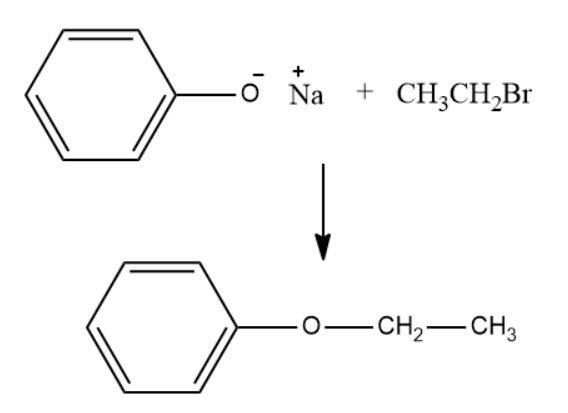Win up to 100% scholarship on Aakash BYJU'S JEE/NEET courses with ABNAT Win up to 100% scholarship on Aakash BYJU'S JEE/NEET courses with ABNAT

# JEE Main 2021 August 27 Shift 1 Chemistry Question Paper

JEE Main 2021 August 27 Shift 1 Chemistry question paper with solutions available here will help JEE aspirants to not only be thorough with the important concepts but also have a quick look at the overall question paper pattern. As students study the JEE Main 2021 session 4 Chemistry question paper, they will get a much clear idea of the paper format, types of questions, marking scheme and the difficulty level of the exam.

### JEE Main 2021 August 27 Shift 1 Chemistry Question Paper

Additionally, the solutions that have been prepared in a precise manner will help students to clearly understand the logic behind solving the different questions present in the paper. With JEE Main 2021 August 27 Shift 1 Chemistry question paper with solutions students will further be able to have a quick revision of the concepts and develop adept time management skills essential for the exam. Students can access the solution along with the JEE Main 2021 Chemistry question paper PDF for August 27 Shift 1 below.

JEE Main 2021 August 27th Shift 1 Chemistry Question Paper

Question 1. In the following equation, find B.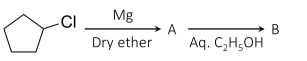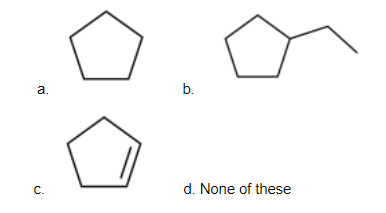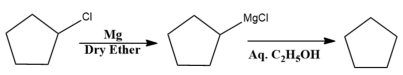Hence, option (a) is the correct answer.

Question 2. A metal having a much low melting point is refined by:

a. Liquation
b. Electrolytic refining
c. Zone Refining
d. None of these

Liquation: In this method, a low melting metal like a tin can be made to flow on a sloping surface. In this way, it is separated from higher melting impurities. This process is used for the purification of Sn and Zn, and for removing Pb from Zn-Ag alloy.

Hence, option (a) is the correct answer.

Question 3. From 0.2 gm of a compound, 0.188 gm of AgBr is formed by Carius Method. Find % of Br?

a. 80%
b. 20%
c. 40%
d. 10%

Molar mass of AgBr = (108 + 80) g/mol = 188 g/mol

188g of AgBr contains 80 g of Br

0.188 g of AgBr will contain 80 x 0.188 / 188 g Br

In 0.2 g of compound % of Br = 80 x 0.188 / 188 x 0.2 x 100% = 40%

Hence, option (c) is the correct answer.

Question 4. In which form uracil is present in DNA?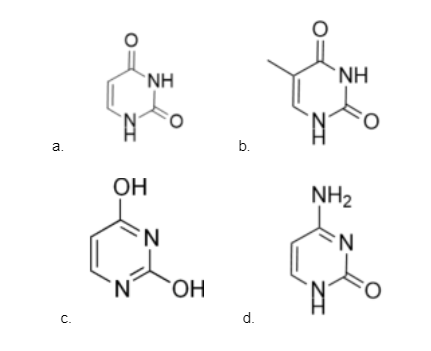Fact-based.

Question 5. Which of the following shows the Tyndall effect?

a. True solution
b. Lyphobic solution
c. Lyophilic solution
d. Suspension

Property

Lyophilic colloids

Lyophobic colloids

Tyndall effect

They do not show the Tyndall effect

They show the Tyndall effect

Hence, option (b) is the correct answer.

Question 6. When the intensity of radiation incident on a photographic plate is increased keeping the frequency constant, then the number and K.E of photoelectrons emitted?

a. Remains same and increases
b. Increases and remains the same
c. Decreases and remains the same
d. Decreases and decreases

As the intensity increases the number of photons striking on the surface increases hence the number of photoelectrons emitted increases whereas the energy of incident photons remains the same and hence the kinetic energy of emitted photoelectrons remains the same.

Hence, option (b) is the correct answer.

Question 7. 0.075 molal solution of sucrose solution containing 1 kg water is at the temperature of – 0.40C, If (Kf)H2O= 1.86 K/m. How much ice will freeze out?

a. 350 g
b. 100 g
c. 180 g
d. 650 g

On freezing moles of sucrose does not change.

ΔTf = i × Kf × m

0.4 = 1 x 1.86 x 0.075 / WH2O x 1000 = 348.75 g

Amount of ice separated= (1000 – 348.75) g

Amount of ice separated = 651.25 ≈ 650 g

Hence, option (d) is the correct answer.

Question 8. One mole of octahedral compound MCl3E2 is reacted with AgNO3 and 1 mol AgCl is formed. Find denticity of E: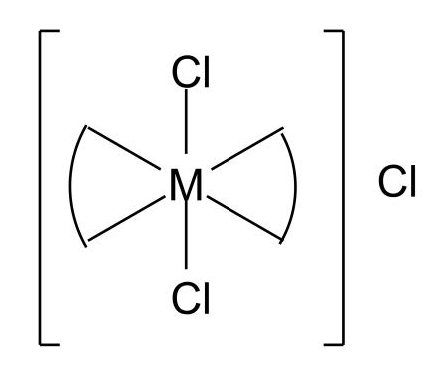One mole of an octahedral compound [MCl3E2] is reacted with AgNO3 to give 1 mol AgCl which means the complex exists as [MCl2E2]Cl. The formation of AgCl is due to the reaction of AgNO3 with Clgiving AgCl. One mole of AgCl is obtained, which means only one chlorine is free. Hence, the complex has to be [MCl2E2]Cl. For an octahedral complex, the coordination number must be 6. Now the denticity of Clis 1 and two Clare present so two valencies are satisfied by chlorine. To make the coordination number 6 ligand E has to be bidentate.

Therefore, the denticity of E must be 2.

Hence, 2 is the correct answer.

Question 9. Find the strength of KMnO4 in gm/L. If 10mL of KMnO4 requires 0.1 M FeSO4 of the same value for decolourisation.

MnO4+ Fe2+ → Mn2+ + Fe3+

Vf = 5 Vf = 1

Milliequivalents of MnO4= Milliequivalents of Fe2+

5 × [M × 10] = 1 × [0.1 × 10]

M = 0.02 x mol / L

Strength of KMnO4 = Molarity × Molar mass

= (0.02 ×158) g/L = 3.16 g/L

Hence, 3.16 is the correct answer.

Question 10. The number of electrons in the outermost f orbital in the ground state of neptunium (Z = 93).

a. 2
b. 3
c. 4
d. 5

Electronic configuration of Neptunium: [Rn]5f4 6d1 7s2

Thus, the number of electrons in the outermost f orbital is 4.

Hence, option (b) is the correct answer.

Question 11. Which of the following is correct with respect to ‘H’ and ‘D’?

a. D produces β+
b. D is more reactive than H
c. H is more reactive than D
d. Equal reactivity

Reactivity of Deuterium is less than hydrogen due to the high bond dissociation energy of D2

Hence, option (c) is the correct answer.

Question 12. Nature of oxides of V2O3 and CrO?

a. Amphoteric and Basic
b. Basic and Basic
c. Basic and Amphoteric
d. Acidic and Acidic

In the case of transition metal oxides, the oxides with metals in lower oxidation states are basic in nature. Since, in V2O3 and CrO, oxidation states of V and Cr are in lower oxidation states i.e., +3 and +2 respectively. Hence both are basic oxides.

In intermediate oxidation states, they are amphoteric in nature. For example, V2O4, CrO2 MnO2 etc. In higher oxidation states, they are acidic in nature. for example, V2O5, CrO3, Mn2O7 etc.

Question 13. Find the oxidation number of ‘S’ in H2S(x+2)O6

a. 0 and +5 only
b. +5 only
c. 0 and +3 only
d. +3 only

When x = 2,

The compound is H2S4O6:

Structure: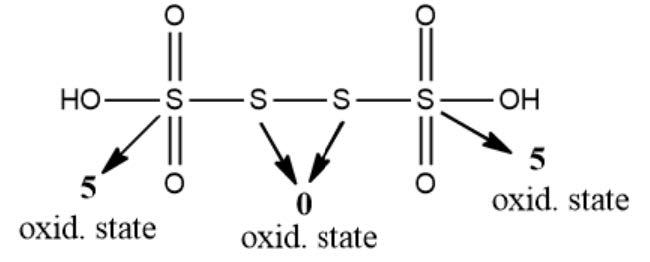So, the oxidation states can be 0 and +5.

Hence, option (c) is the correct answer.

Question 14. Match the following:

Sl. no

Compounds

Number of lone pairs on central atom

1

XeF2

(i)

0

2

XeF2O2

(ii)

1

3

XeF2O3

(iii)

2

4

XeF4

(Iv)

3

a. 1-(i), 2-(iv), 3-(ii), 4-(iii)
b. 1-(iv), 2-(ii), 3-(i), 4-(iii)
c. 1-(iv), 2-(ii), 3-(iii), 4-(i)
d. 1-(iv), 2-(iii), 3-(i), 4-(ii)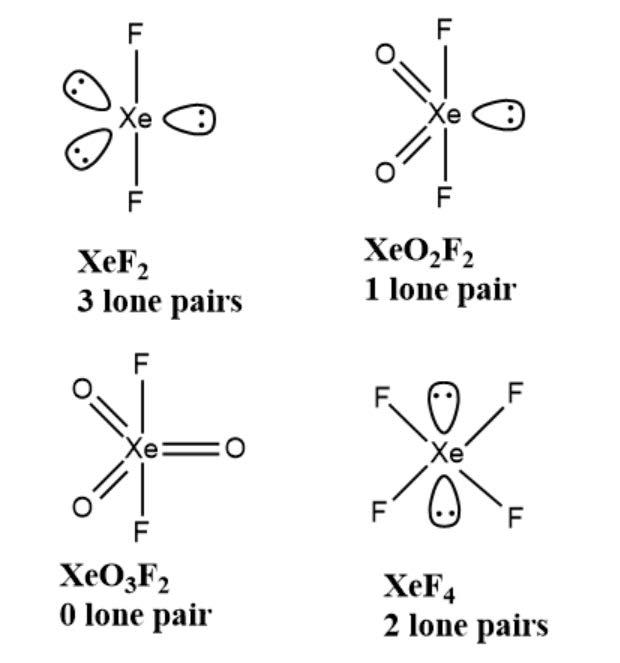Hence, option (b) is the correct answer.

Question 15. Find the incorrect statement regarding primary aliphatic amines:

a. They can be produced by Gabriel phthalimide process
b. Its solubility is greater than 20 amines
c. Can be distinguished by carbylamine test
d. It is less basic than aromatic amines

Gabriel Phthalimide Synthesis is used to get primary amines from primary alkyl halides. Since primary amines have a higher number of hydrogens than secondary amines, they show more H-bonding and hence are more soluble than secondary amines. Primary amines can be distinguished from secondary and tertiary amines by reacting with. Primary amine reacts with CHCl3 and alc. KOH to form isocyanide while secondary and tertiary amines do not react.

In aromatic amines, lone pair of nitrogen is involved in resonance and hence less available for donation. So, aromatic amines are less basic than primary amines.

Hence, option (d) is the correct answer.

Question 16. A reacts with B to form a Prussian blue compound Fe4[Fe(CN)6]3. A and B are respectively:

a. FeCl3 and K3[Fe(CN)6]
b. FeCl3 and K4[Fe(CN)6]
c. K3[Fe(CN)6] and K4[Fe(CN)6]
d. FeCl3 and KCNS

FeCl3 + K4[Fe(CN)6] → Fe4[Fe(CN)6]3

A B Blue colour

Hence, option (b) is the correct answer.

Question 17. Find the order of Lewis acid strength of BI3, BCl3, BF3, BBr3.

a. BF3 < BCl3 < BBr3 < BI3
b. BF3 < BBr3 < BI3 < BCl3
c. BCl3 < BF3 < BBr3 < BI3
d. BBr3 < BF3 < BI3 < BCl3

On the basis of back bonding, the smaller the halide atom, the more effective be the back bonding in BX3.

More effective back bonding shows less tendency to accept a pair of electrons. Hence, correct order of Lewis acid for boron halide is BF3 < BCl3 < BBr3 < BI3.

Question 18. If we have two particles in a system i.e. A & B where particle ‘A’ has 4 amu mass and 2- unit charge and another particle B has 9 amu mass and 3-unit charge. Find the correct option regarding A and B:

a. Deviation of A is more than B
b. Deviation of B is more than A
c. None of them will deviate
d. Same deviation

The deviation of the particle depends on its mass to charge ratio. In general, higher mass to charge ratio particles will deviate less. Here, the mass to charge ratio of A is 2 and the mass to charge ratio of B is 3. Hence, A will deviate more than B.

Question 19. The van der Waal equation is given as:

$$\begin{array}{l}\left ( P + \frac{an^{2}}{V^{2}} \right )(v-nb) = nRT\end{array}$$

a. [atm][m6][mol]-2
b. [atm]2[m2][mol]2
c. [atm]3[m3][mol]1
d. [atm]0

Since,

$$\begin{array}{l}\left ( P + \frac{an^{2}}{V^{2}} \right )\end{array}$$
is a summation, the component P and
$$\begin{array}{l}\frac{an^{2}}{V^{2}}\end{array}$$
should have same dimension.

$$\begin{array}{l}\frac{an^{2}}{V^{2}}\end{array}$$
= [atm]
$$\begin{array}{l}\frac{a(mol)^{2}}{(m^{3})^{2}}\end{array}$$
= [atm]

Hence, dimension of ‘a’ has to be [atm][m6][mol]-2.

Question 20. Find the number of water molecules in gypsum, dead burnt plaster, and Plaster of Paris.

a. 3, 0, 1
b. 1, 0, 1
c. 2, 0, 0.5
d. 5, 0, 0.5

Gypsum = CaSO4. 2H2O

Plaster of Paris = CaSO4.½ H2O

Hence, option (c) is correct.

Question 21. Given below are two statements:

Statement 1: Ethyl phenyl ether can be prepared by Williamson ether synthesis.

Statement 2: Bromobenzene on reacting with sodium ethoxide gives ethyl phenyl ether.

a. Statement I is correct and Statement II is correct
b. Statement I is correct and Statement II is incorrect
c. Statement I is incorrect and Statement II is incorrect
d. Statement I is incorrect and Statement II is correct